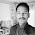## Wednesday, 26 March 2014

### An Artificial Intelligence for the 2048 game

Lately the 2048 game reached a good notoriety on the internet.

A discussion about possible algorithms which solve the 2048 game arose on StackOverflow.

The main discussed algorithms are:

The solution I propose is very simple and easy to implement. Although, it has reached the score of 131040. Several benchmarks of the algorithm performances are presented.

### Algorithm

#### Heuristic scoring algorithm

The assumption on which my algorithm is based is rather simple: if you want to achieve higher score, the board must be kept as tidy as possible. In particular, the optimal setup is given by a linear and monotonic decreasing order of the tile values.

This intuition will give you also the upper bound for a tile value: $2^{n} \rightarrow 2^{16} = 65536$ where $n$ is the number of tile on the board. (There's a possibility to reach the 131072 tile if the 4-tile is randomly generated instead of the 2-tile when needed)

Two possible ways of organizing the board are shown in the following images

To enforce the ordination of the tiles in a monotonic decreasing order, the score si computed as the sum of the linearized values on the board multiplied by the values of a geometric sequence with common ratio $r<1$ .

$p_n \in Path_{0 \cdots N-1}$

$score = \sum_{n=0}^{N-1} value(p_n) * r^n$

Several linear path could be evaluated at once, the final score will be the maximum score of any path.

#### Decision rule

The decision rule implemented is not quite smart, the code in Python is presented here:

An implementation of the minmax or the Expectiminimax will surely improve the algorithm. Obviously a more
sophisticated decision rule will slow down the algorithm and it will require some time to be implemented.I will try a minimax implementation in the near future. (stay tuned)

### Benchmark

• T1 - 121 tests - 8 different paths - $r = 0.125$
• T2 - 122 tests - 8-different paths - $r = 0.25$
• T3 - 132 tests - 8-different paths - $r = 0.5$
• T4 - 211 tests - 2-different paths - $r = 0.125$
• T5 - 274 tests - 2-different paths - $r = 0.25$
• T6 - 211 tests - 2-different paths - $r = 0.5$

In case of T2, four tests in ten generate the 4096 tile with an average score of $\sim 42000$

### Code

The code can be found on GiHub at the following link: https://github.com/Nicola17/term2048-AI
It is based on term2048 and it's written in Python. I will implement a more efficient version in C++ as soon as possible.

### StackOverflow

You can upvote my answer on StackOverflow here :)

1.2048 cupcakes reverse game is a alteration of 2048 game designed and developed by Italian Programmer.

2.Attend The Artificial Intelligence course From ExcelR. Practical Artificial Intelligence course Sessions With Assured Placement Support From Experienced Faculty. ExcelR Offers The Artificial Intelligence course.
Artificial Intelligence course

3.Really Great Post & Thanks for sharing.

Oflox Is The Best Website Design Company In Dehradun

4.5.